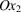International
Tables for
Crystallography
Volume D
Physical properties of crystals
Edited by A. Authier

International Tables for Crystallography (2006). Vol. D, ch. 1.1, p. 17

## Section 1.1.4.7.6. Symmetric tensors of rank 2

A. Authiera*

aInstitut de Minéralogie et de la Physique des Milieux Condensés, Bâtiment 7, 140 rue de Lourmel, 75015 Paris, France
Correspondence e-mail: aauthier@wanadoo.fr

#### 1.1.4.7.6. Symmetric tensors of rank 2

| top | pdf |

If the tensor is symmetric, the number of independent components is still reduced. One obtains the following, representing the nonzero components for the leading diagonal and for one half of the others.

#### 1.1.4.7.6.1. Triclinic system

| top | pdf |There are 6 independent components. It is possible to interpret the number of independent components of a tensor of rank 2 by considering the associated quadric, for instance the optical indicatrix. In the triclinic system, the quadric is any quadric. It is characterized by six parameters: the lengths of the three axes and the orientation of these axes relative to the crystallographic axes.

#### 1.1.4.7.6.2. Monoclinic system (twofold axis parallel to)

| top | pdf |There are 4 independent components. The quadric is still any quadric, but one of its axes coincides with the twofold axis of the monoclinic lattice. Four parameters are required: the lengths of the axes and one angle.

#### 1.1.4.7.6.3. Orthorhombic system

| top | pdf |There are 3 independent components. The quadric is any quadric, the axes of which coincide with the crystallographic axes. Only three parameters are required.

#### 1.1.4.7.6.4. Trigonal, tetragonal and hexagonal systems, isotropic groups

| top | pdf |There are 2 independent components. The quadric is of revolution. It is characterized by two parameters: the lengths of its two axes.

#### 1.1.4.7.6.5. Cubic system

| top | pdf |There is 1 independent component. The associated quadric is a sphere.